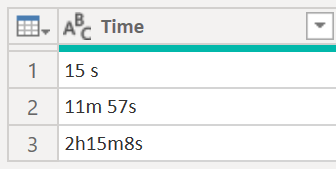top of page
Search
• Antriksh Sharma

# Convert Hour Minute Seconds text to Minutes in Power Query

In this post let's take a look at how to convert a text string such as 2h15m8s to 135.13 minutes

This is the table that we are working with:You can generate it with this code:

```let
Source = Table.FromRows(Json.Document(Binary.Decompress(Binary.FromText("i45WMjRVKFaK1QEyDHMVTM0hbKMMQ9NcCyA7FgA=", BinaryEncoding.Base64), Compression.Deflate)), let _t = ((type nullable text) meta [Serialized.Text = true]) in type table [Time = _t]),

ChangedType = Table.TransformColumnTypes ( Source, { { "Time", type text } } ),

```

Next we will add a new column where we perform the transformation, the first thing is to remove the spaces if any.

```AddedCustom =
ChangedType,
"Total Minutes",
each
let
RemoveSpace = Text.Replace ( [Time], " ", "" ), ```

Once the spaces are removed we can split the text by using Splitter.SplitTextByCharacterTransition and we can split from Text to Numbers.

`Split = Splitter.SplitTextByCharacterTransition ( { "a" .. "z" }, { "0" .. "9" } ) ( RemoveSpace ),`

This step creates a list that looks like this:Once we have this list we can use List.Transform and make some adjustments.

First we can retrieve the Number from each row and check if the current value contains "h" then we multiply by 60 if it contains an "m" then we multiply by 1 else if it contains "s" then we divide by 60.

```Transform =
List.Transform (
Split,
each
let
Number = Number.From (
Text.Select ( _, { "0" .. "9" } )
),
Correction =
if Text.Contains ( _, "h" ) then Number * 60
else if Text.Contains ( _, "m" ) then Number * 1
else if Text.Contains ( _, "s" ) then Number / 60
else null
in
Correction
)```

Finally we can sum this list using List.Sum and Round the number to 2 decimal places.Complete code:

```AddedCustom = Table.AddColumn (
ChangedType,
"Total Minutes",
each
let
RemoveSpace = Text.Replace ( [Time], " ", "" ),
Split =
Splitter.SplitTextByCharacterTransition (
{ "a" .. "z" }, { "0" .. "9" }
) ( RemoveSpace ),
Transform =
List.Transform (
Split,
each
let
Number = Number.From (
Text.Select ( _, { "0" .. "9" } )
),
Correction =
if Text.Contains ( _, "h" ) then
Number * 60
else if Text.Contains ( _, "m" ) then
Number * 1
else if Text.Contains ( _, "s" ) then
Number / 60
else null
in
Correction
),
Sum =  Number.Round ( List.Sum ( Transform ), 2 )
in
Sum,
type number
)```

Here is another way to solve this and probably easier as well. What if instead of splitting and then selecting and multiplying we simpley convert the string into something that represents the calculation but is a text string? And later we can pair Expression.Evaluate with it.Pay attention to the column Minutes, we have a text string that represents mathematical calculation, now all we need to to do is evaluate that.And there you go Expression.Evaluate returns the correct result!